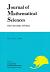## Vol. 15 (2008) No. 02Journal of Mathematical Sciences The University of Tokyo

1. Sasaki, Takeshi; Yoshida, Masaaki
Hyperbolic Schwarz maps of the Airy and the confluent hypergeometric differential equations and their asymptotic behaviors
Vol. 15 (2008), No. 2, Page 195--218.

2. Morita, Kazuma
Galois cohomology of a $p$-adic field via $(\Phi,\Gamma)$-modules in the imperfect residue field case
Vol. 15 (2008), No. 2, Page 219--241.

3. Fejzullahu, Bujar Xh.
A Cohen type inequality for polynomial expansions associated with the measure $(1-x)^\alpha (1+x)^\beta dx+M\delta_{-1}+N\delta_1$}
Vol. 15 (2008), No. 2, Page 243--255.